$\newcommand{\NN}{\mathbb{N}} \newcommand{\CC}{\mathbb{C}} \newcommand{\GG}{\mathbb{G}} \newcommand{\LL}{\mathbb{L}} \newcommand{\PP}{\mathbb{P}} \newcommand{\QQ}{\mathbb{Q}} \newcommand{\RR}{\mathbb{R}} \newcommand{\VV}{\mathbb{V}} \newcommand{\ZZ}{\mathbb{Z}} \newcommand{\FF}{\mathbb{F}} \newcommand{\KK}{\mathbb{K}} \newcommand{\UU}{\mathbb{U}} \newcommand{\EE}{\mathbb{E}} \newcommand{\Aa}{\mathcal{A}} \newcommand{\Bb}{\mathcal{B}} \newcommand{\Cc}{\mathcal{C}} \newcommand{\Dd}{\mathcal{D}} \newcommand{\Ee}{\mathcal{E}} \newcommand{\Ff}{\mathcal{F}} \newcommand{\Gg}{\mathcal{G}} \newcommand{\Hh}{\mathcal{H}} \newcommand{\Ii}{\mathcal{I}} \newcommand{\Jj}{\mathcal{J}} \newcommand{\Kk}{\mathcal{K}} \newcommand{\Ll}{\mathcal{L}} \newcommand{\Mm}{\mathcal{M}} \newcommand{\Nn}{\mathcal{N}} \newcommand{\Oo}{\mathcal{O}} \newcommand{\Pp}{\mathcal{P}} \newcommand{\Qq}{\mathcal{Q}} \newcommand{\Rr}{\mathcal{R}} \newcommand{\Ss}{\mathcal{S}} \newcommand{\Tt}{\mathcal{T}} \newcommand{\Uu}{\mathcal{U}} \newcommand{\Vv}{\mathcal{V}} \newcommand{\Ww}{\mathcal{W}} \newcommand{\Xx}{\mathcal{X}} \newcommand{\Yy}{\mathcal{Y}} \newcommand{\Zz}{\mathcal{Z}} \newcommand{\al}{\alpha} \newcommand{\la}{\lambda} \newcommand{\ga}{\gamma} \newcommand{\Ga}{\Gamma} \newcommand{\La}{\Lambda} \newcommand{\Si}{\Sigma} \newcommand{\si}{\sigma} \newcommand{\be}{\beta} \newcommand{\de}{\delta} \newcommand{\De}{\Delta} \renewcommand{\phi}{\varphi} \renewcommand{\th}{\theta} \newcommand{\om}{\omega} \newcommand{\Om}{\Omega} \renewcommand{\epsilon}{\varepsilon} \newcommand{\Calpha}{\mathrm{C}^\al} \newcommand{\Cbeta}{\mathrm{C}^\be} \newcommand{\Cal}{\text{C}^\al} \newcommand{\Cdeux}{\text{C}^{2}} \newcommand{\Cun}{\text{C}^{1}} \newcommand{\Calt}{\text{C}^{#1}} \newcommand{\lun}{\ell^1} \newcommand{\ldeux}{\ell^2} \newcommand{\linf}{\ell^\infty} \newcommand{\ldeuxj}{{\ldeux_j}} \newcommand{\Lun}{\text{\upshape L}^1} \newcommand{\Ldeux}{\text{\upshape L}^2} \newcommand{\Lp}{\text{\upshape L}^p} \newcommand{\Lq}{\text{\upshape L}^q} \newcommand{\Linf}{\text{\upshape L}^\infty} \newcommand{\lzero}{\ell^0} \newcommand{\lp}{\ell^p} \renewcommand{\d}{\ins{d}} \newcommand{\Grad}{\text{Grad}} \newcommand{\grad}{\text{grad}} \renewcommand{\div}{\text{div}} \newcommand{\diag}{\text{diag}} \newcommand{\pd}{ \frac{ \partial #1}{\partial #2} } \newcommand{\pdd}{ \frac{ \partial^2 #1}{\partial #2^2} } \newcommand{\dotp}{\langle #1,\,#2\rangle} \newcommand{\norm}{|\!| #1 |\!|} \newcommand{\normi}{\norm{#1}_{\infty}} \newcommand{\normu}{\norm{#1}_{1}} \newcommand{\normz}{\norm{#1}_{0}} \newcommand{\abs}{\vert #1 \vert} \newcommand{\argmin}{\text{argmin}} \newcommand{\argmax}{\text{argmax}} \newcommand{\uargmin}{\underset{#1}{\argmin}\;} \newcommand{\uargmax}{\underset{#1}{\argmax}\;} \newcommand{\umin}{\underset{#1}{\min}\;} \newcommand{\umax}{\underset{#1}{\max}\;} \newcommand{\pa}{\left( #1 \right)} \newcommand{\choice}{ \left\{ \begin{array}{l} #1 \end{array} \right. } \newcommand{\enscond}{ \left\{ #1 \;:\; #2 \right\} } \newcommand{\qandq}{ \quad \text{and} \quad } \newcommand{\qqandqq}{ \qquad \text{and} \qquad } \newcommand{\qifq}{ \quad \text{if} \quad } \newcommand{\qqifqq}{ \qquad \text{if} \qquad } \newcommand{\qwhereq}{ \quad \text{where} \quad } \newcommand{\qqwhereqq}{ \qquad \text{where} \qquad } \newcommand{\qwithq}{ \quad \text{with} \quad } \newcommand{\qqwithqq}{ \qquad \text{with} \qquad } \newcommand{\qforq}{ \quad \text{for} \quad } \newcommand{\qqforqq}{ \qquad \text{for} \qquad } \newcommand{\qqsinceqq}{ \qquad \text{since} \qquad } \newcommand{\qsinceq}{ \quad \text{since} \quad } \newcommand{\qarrq}{\quad\Longrightarrow\quad} \newcommand{\qqarrqq}{\quad\Longrightarrow\quad} \newcommand{\qiffq}{\quad\Longleftrightarrow\quad} \newcommand{\qqiffqq}{\qquad\Longleftrightarrow\qquad} \newcommand{\qsubjq}{ \quad \text{subject to} \quad } \newcommand{\qqsubjqq}{ \qquad \text{subject to} \qquad }$

Wavelet Compression of Integral Operators

# Wavelet Compression of Integral Operators

This numerical tour explores approximation of integral operators over wavelets bases.

## Installing toolboxes and setting up the path.

You need to download the following files: signal toolbox and general toolbox.

You need to unzip these toolboxes in your working directory, so that you have toolbox_signal and toolbox_general in your directory.

For Scilab user: you must replace the Matlab comment '%' by its Scilab counterpart '//'.

Recommandation: You should create a text file named for instance numericaltour.sce (in Scilab) or numericaltour.m (in Matlab) to write all the Scilab/Matlab command you want to execute. Then, simply run exec('numericaltour.sce'); (in Scilab) or numericaltour; (in Matlab) to run the commands.

Execute this line only if you are using Matlab.

getd = @(p)path(p,path); % scilab users must *not* execute this


Then you can add the toolboxes to the path.

getd('toolbox_signal/');
getd('toolbox_general/');


## Representation of Operators in Bases

We consider 1-D signals $$f \in \Hh=L^2([0,1])$$ (we assume periodic boundary conditions). We consider an orthogonal basis $$\Bb = (\psi_m)_{m \in \Om}$$ of $$\Hh$$. The forward (decomposition) operator is $\Psi : f \in \Hh \rightarrow (\dotp{f}{\psi_m})_{m \in \Om} \in \Vv = \RR^\Om.$ The backaward (recomposition) operator is $\Psi^* : c \in \Vv \rightarrow \sum_{m \in \Om} c_m \psi_m \in \Hh.$

Note that $$\Bb$$ being orthonormal is equivalent to $\Psi^* \circ \Psi = \text{Id}_\Hh \qandq \Psi \circ \Psi^* = \text{Id}_\Vv.$

A linear operator $$T : \Hh \rightarrow \Hh$$ can be represented as $T f(x) = \int_0^1 K(x,y) f(y) d y.$

Here $$K : [0,1]^2 \rightarrow \RR$$ is a kernel function, that is sometimes assumed to be a distribution (for instance to define differential operators).

$$K$$ is in some sense the representation of $$T$$ in the Dirac basis of $$\Hh$$. One can represent the operator $$T$$ in the basis $$\Bb$$ as follow $T = \Psi^* S \Psi \qwhereq S = \Psi T \Psi^* \in \ell^2(\Vv).$ Here the operator $$S : \Vv \rightarrow \Vv$$ can be understood as an (possibly infinite) matrix whose entries can be computed as $\forall (m,m') \in \Omega^2, \quad S_{m,m'} = \dotp{T \psi_m}{\psi_{m'}}.$

In the following, in order to be able to perform numerical computations, we consider discretized signals $$f \in \Hh = \RR^N$$ and thus assume $$\Psi$$ defines an orthonormal basis of $$\RR^N$$. In this case $$\Vv = \RR^N$$, and both $$K, S \in \RR^{N \times N}$$.

The switch of representations $\Theta : T \in \RR^{N \times N} \longmapsto S = \Psi T \Psi^* \in \RR^{N \times N}$ is called the tensorial transform assiociated to $$\Psi$$ (also called the anisotropic transform in the case of Wavelet bases).

It can be computed numerically by first applying $$\Psi$$ to the rows of $$T$$ and then to its columns.

## Foveation Operator

We consider here a special case of integral operator, the so-called Foveation operator. It corresponds to a spacially varying convolution.

Size $$N$$ of the signal.

N = 1024;


We can compute a signal and the action of the kernel on the signal.

f = load_signal('piece-polynomial', N);


Display it.

clf;
plot(f); axis tight;A spacially varying convolution is a blurring operator that is not translation invariant. For a Foveation operator, the blur has a spatially varying bandwidth parameterized by a scale $$\si(x)$$, that dillates a given convolution profile $$\phi(x)$$. It thus corresponds to $K(x,y) = \frac{1}{\si(x)} \phi\pa{ \frac{x-y}{\si(x)} }.$

We choose here a Gaussian kernel.

phi = @(x)exp( -abs(x).^2 / 2 );


One usually chose a foveation scale that increases linearly with the distance to some center point $$c$$ of Foveation. This reproduces the effect of human vision, that has a finer resolution in the center of the field of view. We thus choose $\si(x) = \al \abs{x-c} + \be$ for some constants $$\al,\be > 0$$.

Center point $$c$$ of foveation

c = 1/2;


Rate of foveation and offset.

alpha = .05;
beta = 1e-3;


Definition of $$\sigma$$

sigma = @(x)alpha*abs(x-c)+beta;


Normalization operator (this is needed for the discrete kernel) the rows.

normalize = @(K)K ./ repmat( sum(K,2), [1 N] );


Definition of the kernel.

t = (0:N-1)/N;
[Y,X] = meshgrid(t,t);
K = normalize( phi( (X-Y) ./ sigma(X)  ) );


Display a few lines of the kernel.

I = round( linspace(1,N,17) );
clf;
plot(K(:,I)); axis tight;
axis([1 N -.005 .1]);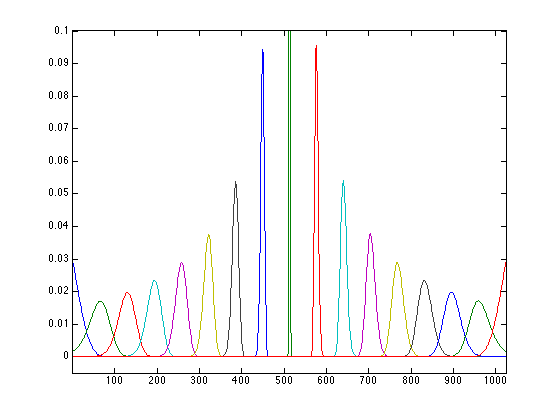Display the kernel as an image (using a change of contrast to better view the rows).

clf;
imageplot(K.^.1);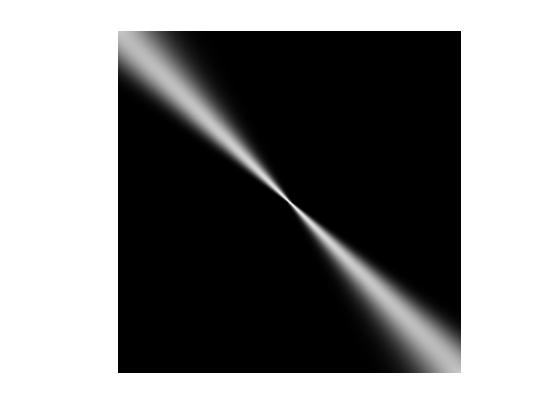Compute $$f = T g$$. In the discrete setting, this corresponds to the multiplication by the discretized kernel $$K$$ (which is the representation of $$T$$ in the Dirac basis).

g = K*f;


Display the action of the kernel.

clf;
subplot(2,1,1);
plot(f); axis('tight');
title('f');
subplot(2,1,2);
plot(g); axis('tight');
title('g');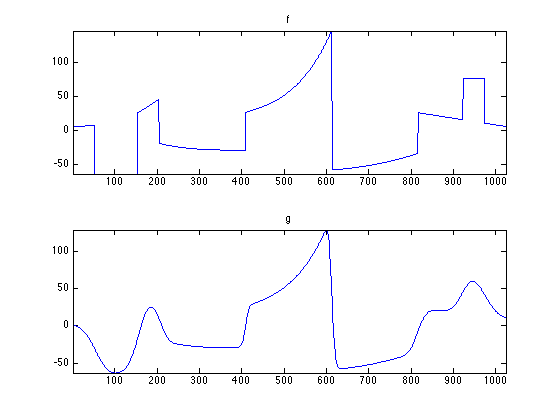For later use, store the average application time.

t0 = 0;
ntrials = 50;
for i=1:ntrials
tic; g = K*f; t0 = t0 + toc();
end
t0 = t0 / ntrials;


## Representation of Operator in a Wavelet Basis

Wavelet operator compression has been initially introduced for the compression of integral operator with a kernel $$K$$ that is sufficiently smooth [BeyCoifRokh91]. In this setting, this allows to reduce the storage of the discretized matrix $$T \in \RR^{N \times N}$$ from $$O(N^2)$$ to $$O(N\log(N))$$ by thresholding the entries of $$S$$.

A more advanced representation, the so-called non-standard one, allows an even further compression to $$O(N)$$ entries. We do not detail here the non-standard representation, that is more involved.

Representation of operator in wavelet bassis has also been applied to local differential operator (in which case the kernel $$K$$ is a distribution), see for instance [Beylkin92]. In this case, the advantage is not the storage of the matrix, but the ability to derive optimal pre-conditioner that are diagonal in the transformed domain, see for instance [DahmKun92]. This allows one to recover and extend classical multiscale preconditonners, such as for instance [BramPascXu90]. Such preconditionners are crucial to solve linear systems such as $$T f = y$$ (which typically corresponds to the resolution of a partial differential equation) using for instance the conjugate gradient method.

An orthogonal wavelet basis of $$\Hh$$ reads $\forall m = (j,n), \quad \psi_{m}(x) = \frac{1}{2^{j/2}} \psi\pa{ \frac{x-2^j n}{2^j} }$ for scales $$j \in \ZZ$$ and positions $$n = 0,\ldots,2^{-j}-1$$.

We use here the simplest wavelet, the Haar wavelets. Wavelet with a larger number of vanishing moments could be used as well.

options.filter = 'haar';
Jmin = 1;


Define the forward transform $$\Psi$$ and the backward transform $$\Psi^*$$.

options.separable = 0;
Psi  = @(f)perform_wavelet_transf(f, Jmin, +1, options);
PsiS = @(f)perform_wavelet_transf(f, Jmin, -1, options);


We define the tensor product transform $\Theta(T) = \Psi T \Psi^*$ where $$T$$ is represented through its kernel $$K$$.

options.separable = 1;
Theta = @(K)perform_wavelet_transf(K, Jmin, +1, options);


## Wavelet Foveation

Computing foveation over wavelet bases has been initially proposed in [ChaMalYap00]. It extends an initial method proposed by [Burt88].

Display the wavelet transform $$\Psi f$$ of the signal $$f$$.

clf;
plot_wavelet(Psi(f), Jmin);compute $$S = \Theta(T)$$.

S = Theta(K);


Display $$S$$.

clf;
plot_wavelet(S, Jmin, options);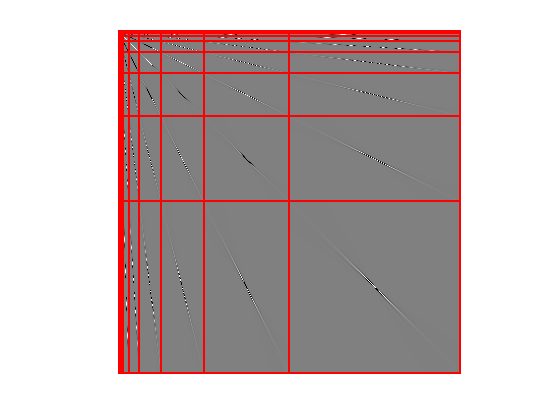The operator is compressed by computing a sparse matrix $$S_\tau$$ that approximate $$S$$. Since most of the entries of $$S$$ are small, one achieve this compression using a non-linear hard thresholding $(S_\tau)_{i,j} = \choice{ S_{i,j} \qifq \abs{S_{i,j}}>\tau, \\ 0 \quad \text{otherwise}. }$ One thus defined $$S_\tau = \Gamma_\tau(S)$$ where $$\Gamma_\tau$$ is the hard thresholding operator.

Gamma = @(S,tau)S .* ( abs(S)>tau );


The threshold $$\tau$$ can be adapted to ensure that $$S_\tau$$ has only $$m$$ non-zero entries. This defines $$\tau=\tau(m)$$.

subs = @(u,m)u(m);
tau = @(m)subs( sort(abs(S(:)), 'descend'), m);


Set the targeted number of coefficients.

m = 4*N;


In order to save computation time when applying $$S_\tau$$, it should be stored as a sparse matrix $$S_1$$.

S1 = sparse(Gamma(S,tau(m)));


Display the support of the $$m$$ largest coefficients that are used for the approximation.

clf;
plot_wavelet(zeros(N), Jmin, options); hold on;
spy(S1);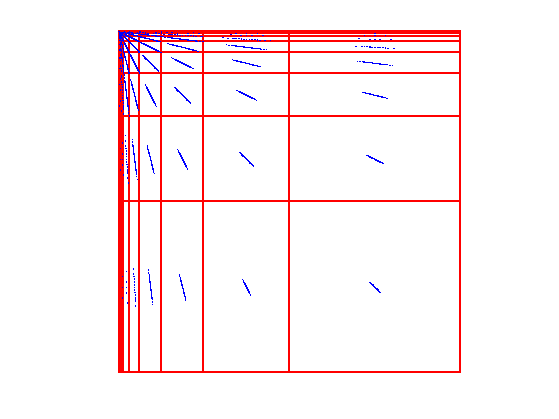One can use this compressed operator to approximate $g = \Psi^* S \Psi f$ by $g_\tau = \Psi^* S_\tau \Psi f.$ When $$\tau \rightarrow 0$$, $$g_\tau \rightarrow g$$. When $$\tau$$ is large, since $$S_\tau$$ is a sparse matrix, computing $$g_\gau$$ is faster than computing $$g$$.

Exercice 1: (check the solution) Display the result $$g_{\tau(m)}$$ for different values of $$m$$.

exo1;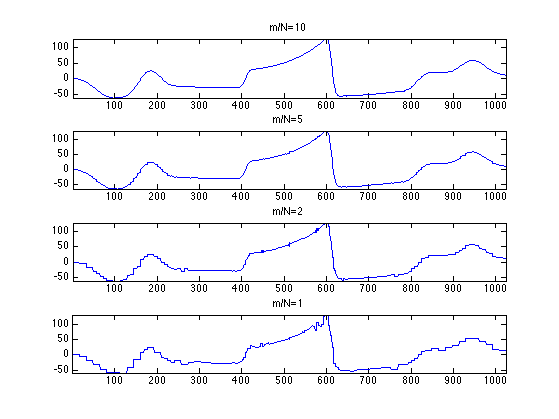Exercice 2: (check the solution) Study the speed gain as a function of $$m$$ of using the sparse multiplication with respect to the direct computation of $$T f$$.

exo2;


Operator compression is not only useful to compute efficiently approximation of product $$T f$$. It can also be used for instance to compute approximation of the inverse $$T^{-1}$$, see for instance [BeyCoifRokh91].